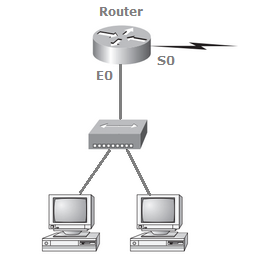# Networking - Subnetting - Discussion

### Discussion :: Subnetting - Subnetting (Q.No.17)

17.

Using the following illustration, what would be the IP address of E0 if you were using the eighth subnet? The network ID is 192.168.10.0/28 and you need to use the last available IP address in the range. The zero subnet should not be considered valid for this question.[A]. 192.168.10.142 [B]. 192.168.10.66 [C]. 192.168.100.254 [D]. 192.168.10.143 [E]. 192.168.10.126

Explanation:

A /28 is a 255.255.255.240 mask. Let's count to the ninth subnet (we need to find the broadcast address of the eighth subnet, so we need to count to the ninth subnet). Starting at 16 (remember, the question stated that we will not use subnet zero, so we start at 16, not 0), 16, 32, 48, 64, 80, 96, 112, 128, 144. The eighth subnet is 128 and the next subnet is 144, so our broadcast address of the 128 subnet is 143. This makes the host range 129-142. 142 is the last valid host.

 Chathu said: (Oct 12, 2015) Please answer this quiz. What is the net work ID, Broadcast ID, Number of Hosts and useful IP range of 168.16.64.0, 255.255.192.0 network block?

 Rcybervirus said: (Oct 24, 2015) In the question its asking for the 8th subnet then according to question the correct option should be (E). But why in explanation it has taken as 9th subnet somebody explain please?

 Manu Ratheesh said: (Nov 22, 2015) @Rcybervirus. Given IP is 192.168.10.0/28 => the SM is 255.255.255.240.0. => NID is 28 bits and HID is remaining 4 bits. So the number of IP's per subnet is 2^4 = 16. 0th subnet-> 0 to 15. 1st -> 16 to 31. 2nd 32 to 47. 3rd 48 to 63. 4th 64 to 79. 5th 80 to 95. 6th 96 to 111. 7th 112 to 127. 8th 128 to 143. In the 8th subnet, the first IP (192.168.10.128) is used as the subnet address and the last IP (192.168.10.143) is used as the broadcast address. It can't be assigned to anyone. So the last IP of the eighth subnet is 192.168.10.142. Option is (A).

 Rajib said: (May 30, 2016) @Manu Ratheesh. Given that SM is 255.255.192.0 => NID is 18 bits and HID is 14 bits. This IP is class B range but you mention NID is 18 and HID are 14 why? I think CLASS B (N.N.H.N = 32)that means NID = 16bit and the HID = 16 bit that's it.

 Manu Ratheesh said: (Jul 4, 2016) @Rajib Total number of bits for network : 16 bits (class B's NID) + 2 (subnet) = 18, So, remaining 14 bits for host id.

 Chandrashekar said: (Aug 4, 2016) @ Manu Ratheesh. 0th subnet-> 0 to 15. 1st -> 16 to 31. 2nd -> 32 to 47. 3rd -> 48 to 63. 4th -> 64 to 79. 5th -> 80 to 95. 6th -> 96 to 111. 7th -> 112 to 127. 8th -> 128 to 143. In question they said the zero subnet shouldn't be considered valid for this question right then the 0th subnet would start from 1 to 16, isn't it?

 Rupesh Wagh said: (Feb 14, 2017) IP Pool = 168.16.64.0, 255.255.192.0 that means (168.16.64.0/18). Answer. IP Pool 168.16.64.0. Subnet(S/M) 255.255.192.0. Start Network IP 168.16.64.0. Broadcast IP is 168.16.127.255.